# solid geometry

2 posts / 0 new
Renz Kolin Gaba...solid geometry

The silo shown in a sketch is an air- and water-tight tower. It consist of a lower cylinder surmounted by a frustum of a cone whose lower base is a upper base of the cylinder. The frustum in turn is surmounted by a cupola consisting of a smaller cylinder whose lower base is the upper base of the frustum. This smaller cylinder is topped by a conical roof. The inside radii of the smaller and larger cylinders are 6 ft. and 12 ft., respectively. The altitudes of the frustum and larger cylinder are 6 ft. and 21 ft., respectively. If ensilage can be stored up to the cupola, find the storage capacity of the silo.

fitzmerl duronTo answer the question above, kailangan natin ng guhit....hehehehehe

It looks like this: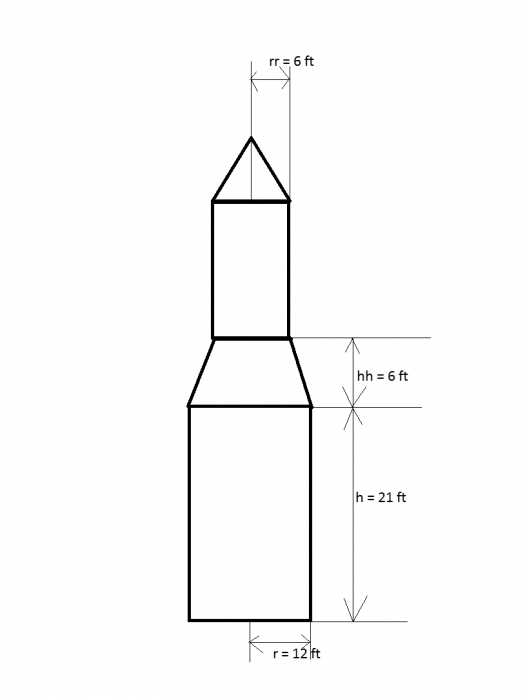To get the volume of the silo above, notice that the silo is a composite solid figure composed of a big cylinder, frustrum of a cone, small cylinder and a cone. Therefore, the volume of the silo is the volume of the big cylinder $+$ volume of the frustrum of a cone $+$ volume of the small cylinder $+$ volume of a cone.

In figures: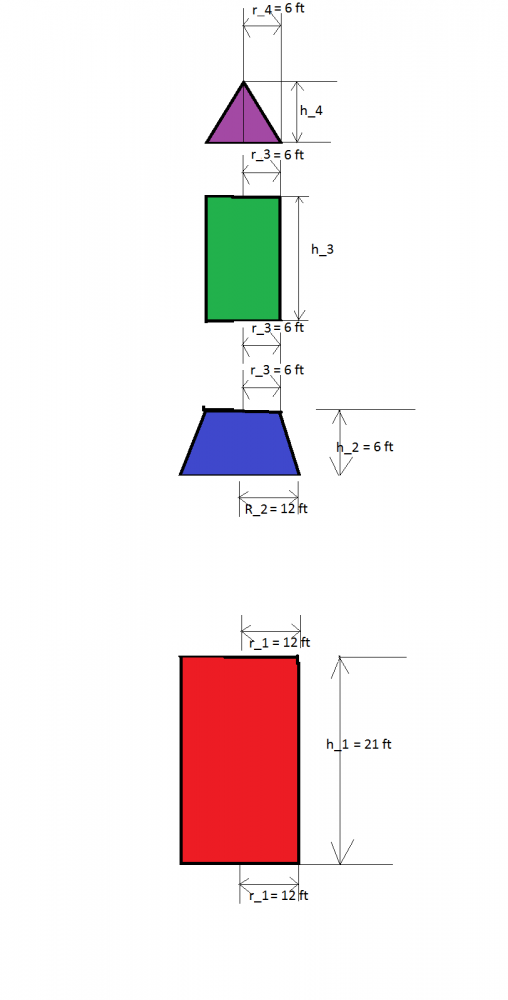$$\mathbf{EQUALS}$$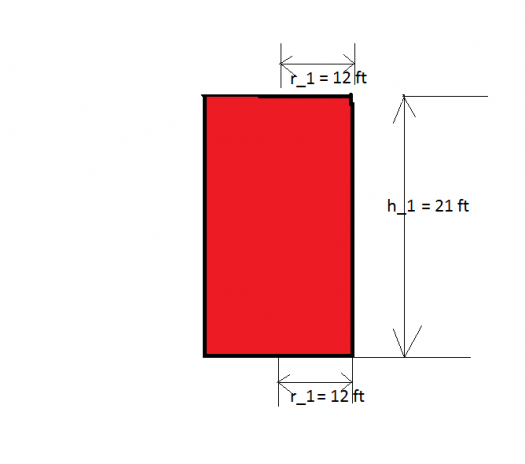$$\mathbf{PLUS}$$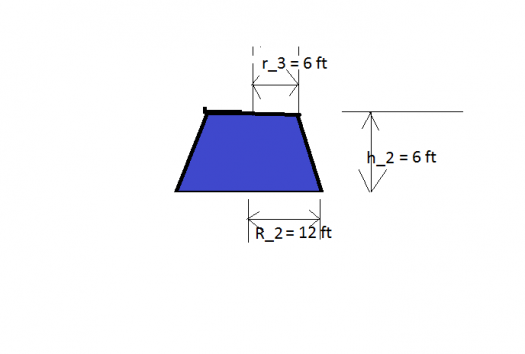$$\mathbf{PLUS}$$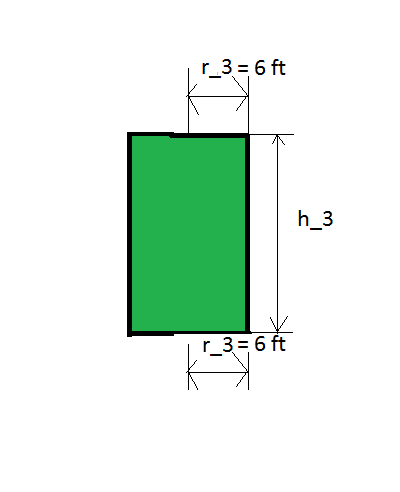$$\mathbf{PLUS}$$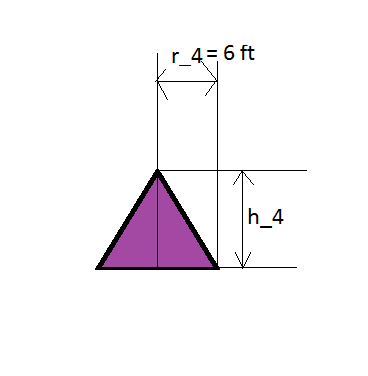$$volume \space of \space the \space silo \space = \color{red}{\space volume \space of \space the \space big \space cylinder \space} + \color{blue}{\space volume \space of \space the \space frustrum \space of \space a \space cone \space} + \color{green}{\space volume \space of \space the \space small \space cylinder \space} + \color{violet}{\space volume \space of \space a \space cone}$$ $$Volume \space of \space silo = \color{red}{\pi r_1^2 h_1} + \color{blue}{\frac{\pi h_2}{3}(R_2^2 + r_3^2 +R_2r_3)} + \color{green}{\pi r_3^2 h_3} + \color{violet}{\frac{1}{3}\pi r_4^2 h^4}$$

Notice that the height $h_3$ is unknown, but we can still get it by remembering what must be the height of cylinder to achieve the largest possible volume of cylinder, given is the radius of its bases. Turns out that the height must be $\mathbf {two \space times}$ longer that the radius of the bases to achieve the cylinder's maximum volume. Height $h_3$, therefore is $6 \times 2$ or $12 \space units$.

Notice that the height $h_4$ is unknown, but we can still get it by remembering what must be the height of cone to achieve the largest possible volume of cone, given is the radius of its base. Turns out that the height must be $\mathbf {\frac{1}{\sqrt{2}} \space times}$ longer that the radius of the base to achieve the cone's maximum volume. Height $h_4$, therefore is $6 \times \frac{1}{\sqrt{2}}$ or $4.2 \space units$. That's one stubby cone.

Therefore, the volume of the silo is:

$$Volume \space of \space silo = \color{red}{\pi r_1^2 h_1} + \color{blue}{\frac{\pi h_2}{3}(R_2^2 + r_3^2 +R_2r_3)} + \color{green}{\pi r_3^2 h_3} + \color{violet}{\frac{1}{3}\pi r_4^2 h^4}$$ $$Volume \space of \space silo = \color{red}{\pi (12)^2 (21)} + \color{blue}{\frac{\pi (6)}{3}((12)^2 + (6)^2 +(12)(6))} + \color{green}{\pi (6)^2 (12)} + \color{violet}{\frac{1}{3}\pi (6)^2 (4.2)}$$ $$Volume \space of \space silo = \color{red}{9500.2 \space ft^3} + \color{blue}{1583.4 \space ft^3} + \color{green}{1357.2 \space ft^3} + \color{violet}{158.3 \space ft^3}$$ $$\color{green}{Volume \space of \space silo = 12599.1 \space ft^3}$$

Therefore, the volume of the silo is $\color{green}{12599.1 \space ft^3}$.

Alternate solutions are encouraged.....

Subscribe to MATHalino on